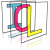Image Component Library (ICL)
icl::math::StochasticOptimizer< T > Class Template Referenceabstract

The StochasticOptimizer is a tiny frame-work for simple stochastic optimization processes. More...

`#include <StochasticOptimizer.h>`

## Public Types

typedef StochasticOptimizerResult< T > Result
Result structure. More...

## Public Member Functions

create a stochastic optimizer with given data dimension More...

Result optimize (int maxTimeSteps)
start optimization process with given step count More...

Result optimize (T minError, int maxSteps)
start optimization process with given step count and minimal error stop-criterion More...

## Protected Member Functions

virtual T * getData ()=0
must return the current data vector More...

virtual T getError (const T *data)=0
must return current error value (>=0) More...

virtual const T * getNoise (int currentTime, int endTime)=0
returns a noise vector (of size dataDim, which was passed to the constructor) More...

virtual void reinitialize ()=0
this function is called before the optimization is started More...

virtual void notifyProgress (int t, int numSteps, int startError, int currBestError, int currError, const T *data, int dataDim)
a pure utility function, which can be implemented in derived classes to notify optization progress (somehow) More...

## Private Attributes

internal data-dimension variable More...

## Detailed Description

### template<class T = float> class icl::math::StochasticOptimizer< T >

The StochasticOptimizer is a tiny frame-work for simple stochastic optimization processes.

Stochastic optimization is minimization of an error function, which depends on a set of latent variables (here: called data). The naive approach origins from starting configuration, which must be created by the pure virtual reinitialize function. Now in each optimization step, the current data vector is changed slightly using an additive noise vector which must be provided by the also pure virtual getNoise-function. If the error arising from the changed data is less then the current minimal error, then the change data vector use hold, otherwise the last change of data is reverted. This procedure is iterated until either a maximum number of iterations is reached or until a given minimal error is reached.

NEW** now this class is a template (defined for float and double)

## ◆ Result

template<class T = float>
 typedef StochasticOptimizerResult icl::math::StochasticOptimizer< T >::Result

Result structure.

## ◆ StochasticOptimizer()

template<class T = float>
 icl::math::StochasticOptimizer< T >::StochasticOptimizer ( int dataDim )

create a stochastic optimizer with given data dimension

## ◆ getData()

template<class T = float>
 virtual T* icl::math::StochasticOptimizer< T >::getData ( )
protectedpure virtual

must return the current data vector

data is un-const, as it is changed in each step if the reached error is less then the current best error. Returned pointer must have at least the length of dataDim passed to the constructor

## ◆ getError()

template<class T = float>
 virtual T icl::math::StochasticOptimizer< T >::getError ( const T * data )
protectedpure virtual

must return current error value (>=0)

returns the error measurement dependent on given data vector

## ◆ getNoise()

template<class T = float>
 virtual const T* icl::math::StochasticOptimizer< T >::getNoise ( int currentTime, int endTime )
protectedpure virtual

returns a noise vector (of size dataDim, which was passed to the constructor)

optionally, the noise-strength might depend on the current time-progress. Therefore, currentTime and endTime is also passed to this functions

## ◆ notifyProgress()

template<class T = float>
 virtual void icl::math::StochasticOptimizer< T >::notifyProgress ( int t, int numSteps, int startError, int currBestError, int currError, const T * data, int dataDim )
protectedvirtual

a pure utility function, which can be implemented in derived classes to notify optization progress (somehow)

## ◆ optimize() [1/2]

template<class T = float>
 Result icl::math::StochasticOptimizer< T >::optimize ( int maxTimeSteps )
inline

start optimization process with given step count

## ◆ optimize() [2/2]

template<class T = float>
 Result icl::math::StochasticOptimizer< T >::optimize ( T minError, int maxSteps )

start optimization process with given step count and minimal error stop-criterion

## ◆ reinitialize()

template<class T = float>
 virtual void icl::math::StochasticOptimizer< T >::reinitialize ( )
protectedpure virtual

this function is called before the optimization is started

Internally data must be initialized